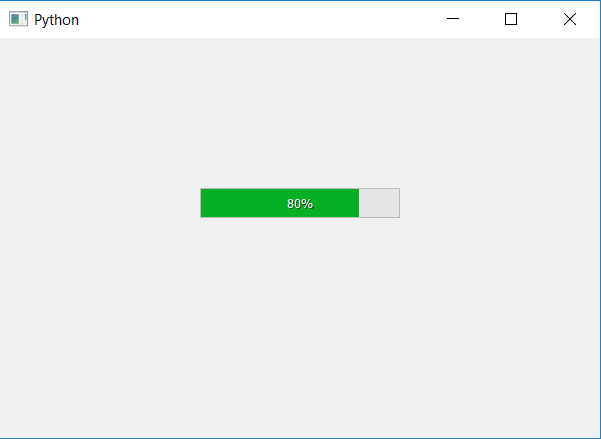# PyQt5 – How to set minimum value of progress bar ?

• Last Updated : 22 Apr, 2020

In this article we will see how to set the minimum value of progress bar. By default the minimum value of progress bar is 0, but we can change it to our need. In order to do this we will use `setMinimum` method, this will change the maximum value of progress bar.

If we change the minimum value, percentage will change accordingly

`percentage = ((value_passed - minimum_value)/(maximum_value - minimum_value))*100`

Here maximum_value = 100, value_passed and minimum_value is set by user.

Syntax : bar.setMinimum(minimum_value)

Argument : It takes integer as argument.

Action performed : It will set the minimum value of progress bar.

Code :

 `# importing libraries``from` `PyQt5.QtWidgets ``import` `*` `from` `PyQt5 ``import` `QtCore, QtGui``from` `PyQt5.QtGui ``import` `*` `from` `PyQt5.QtCore ``import` `*` `import` `sys`` ` ` ` `class` `Window(QMainWindow):`` ` ` ` `    ``def` `__init__(``self``):``        ``super``().__init__()`` ` `        ``# setting title``        ``self``.setWindowTitle(``"Python "``)`` ` `        ``# setting geometry``        ``self``.setGeometry(``100``, ``100``, ``600``, ``400``)`` ` `        ``# calling method``        ``self``.UiComponents()`` ` `        ``# showing all the widgets``        ``self``.show()`` ` `    ``# method for widgets``    ``def` `UiComponents(``self``):`` ` `        ``# creating progress bar``        ``bar ``=` `QProgressBar(``self``)`` ` `        ``# setting geometry to progress bar``        ``bar.setGeometry(``200``, ``150``, ``200``, ``30``)`` ` `        ``# setting minimum value of progress bar to 50``        ``bar.setMinimum(``50``)`` ` `        ``# setting value to progress bar``        ``bar.setValue(``90``)`` ` `        ``# setting alignment to centre``        ``bar.setAlignment(Qt.AlignCenter)`` ` ` ` `# create pyqt5 app``App ``=` `QApplication(sys.argv)`` ` `# create the instance of our Window``window ``=` `Window()`` ` `# start the app``sys.exit(App.``exec``())`

Output :My Personal Notes arrow_drop_up